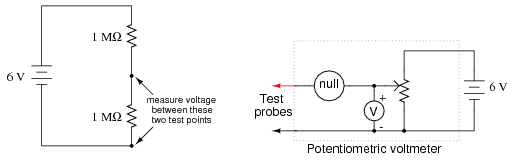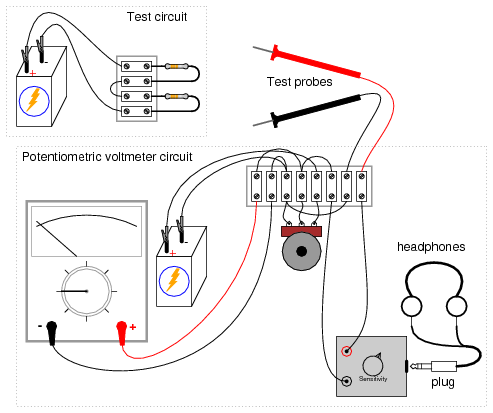# 3.13: Potentiometric Voltmeter

PARTS AND MATERIALS

• Two 6 volt batteries
• One potentiometer, single turn, 10 kΩ, linear taper (Radio Shack catalog # 271-1715)
• Two high-value resistors (at least 1 MΩ each)
• Sensitive voltage detector (from the previous experiment)
• Analog voltmeter (from the previous experiment)

The potentiometer value is not critical: anything from 1 kΩ to 100 kΩ is acceptable. If you have built the “precision potentiometer” described earlier in this chapter, it is recommended that you use it in this experiment.

Likewise, the actual values of the resistors are not critical. In this particular experiment, the greater the value, the better the results. They need not be precisely equal value, either.

If you have not yet built the sensitive voltage detector, it is recommended that you build one before proceeding with this experiment! It is a very useful, yet simple, a piece of test equipment that you should not be without. You can use a digital multimeter set to the “DC millivolt” (DC mV) range in lieu of a voltage detector, but the headphone-based voltage detector is more appropriate because it demonstrates how you can make precise voltage measurements without using expensive or advanced meter equipment. I recommend using your home-made multimeter for the same reason, although any voltmeter will suffice for this experiment.

CROSS-REFERENCES

Lessons In Electric Circuits, Volume 1, chapter 8: “DC Metering Circuits”

LEARNING OBJECTIVES

• Using a potentiometer as a source of variable voltage
• Potentiometric method of voltage measurement

SCHEMATIC DIAGRAMILLUSTRATIONINSTRUCTIONS

Build the two-resistor voltage divider circuit shown on the left of the schematic diagram and of the illustration. If the two high-value resistors are of equal value, the battery’s voltage should be split in half, with approximately 3 volts dropped across each resistor.

Measure the battery voltage directly with a voltmeter, then measure each resistor’s voltage drop. Do you notice anything unusual about the voltmeter’s readings? Normally, series voltage drops add to equal the total applied voltage, but in this case, you will notice a serious discrepancy. Is Kirchhoff’s Voltage Law untrue? Is this an exception to one of the most fundamental laws of electric circuits? No! What is happening is this: when you connect a voltmeter across either resistor, the voltmeter itself alters the circuit so that the voltage is not the same as with no meter connected.

I like to use the analogy of an air pressure gauge used to check the pressure of a pneumatic tire. When a gauge is connected to the tire’s fill valve, it releases some air out of the tire. This affects the pressure in the tire, and so the gauge reads a slightly lower pressure than what was in the tire before the gauge was connected. In other words, the act of measuring tire pressure alters the tire’s pressure. Hopefully, though, there is so little air released from the tire during the act of measurement that the reduction in pressure is negligible. Voltmeters similarly impact the voltage they measure, by bypassing some current around the component whose voltage drop is being measured. This affects the voltage drop, but the effect is so slight that you usually don’t notice it.

In this circuit, though, the effect is very pronounced. Why is this? Try replacing the two high-value resistors with two of 100 kΩ value each and repeat the experiment. Replace those resistors with two 10 KΩ units and repeat. What do you notice about the voltage readings with lower-value resistors? What does this tell you about voltmeter “impact” on a circuit in relation to that circuit’s resistance? Replace any low-value resistors with the original, high-value (>= 1 MΩ) resistors before proceeding.

Try measuring voltage across the two high-value resistors—one at a time—with a digital voltmeter instead of an analog voltmeter. What do you notice about the digital meter’s readings versus the analog meter’s? Digital voltmeters typically have greater internal (probe-to-probe) resistance, meaning they draw less current than a comparable analog voltmeter when measuring the same voltage source. An ideal voltmeter would draw zero current from the circuit under test, and thus suffer no voltage “impact” problems.

If you happen to have two voltmeters, try this: connect one voltmeter across one resistor, and the other voltmeter across the other resistor. The voltage readings you get will add up to the total voltage this time, no matter what the resistor values are, even though they’re different from the readings obtained from a single meter used twice. Unfortunately, though, it is unlikely that the voltage readings obtained this way are equal to the true voltage drops with no meters connected, and so it is not a practical solution to the problem.
Is there any way to make a “perfect” voltmeter: one that has infinite resistance and draws no current from the circuit under test? Modern laboratory voltmeters approach this goal by using semiconductor “amplifier” circuits, but this method is too technologically advanced for the student or hobbyist to duplicate. A much simpler and much older technique is called the potentiometric or null-balance method. This involves using an adjustable voltage source to “balance” the measured voltage. When the two voltages are equal, as indicated by a very sensitive null detector, the adjustable voltage source is measured with an ordinary voltmeter. Because the two voltage sources are equal to each other, measuring the adjustable source is the same as measuring across the test circuit, except that there is no “impact” error because the adjustable source provides any current needed by the voltmeter. Consequently, the circuit under test remains unaffected, allowing measurement of its true voltage drop.

Examine the following schematic to see how the potentiometric voltmeter method is implemented:The circle symbol with the word “null” written inside represents the null detector. This can be any arbitrarily sensitive meter movement or voltage indicator. Its sole purpose in this circuit is to indicate when there is zero voltage: when the adjustable voltage source (potentiometer) is precisely equal to the voltage drop in the circuit under test. The more sensitive this null detector is, the more precisely the adjustable source may be adjusted to equal the voltage under test, and the more precisely that test voltage may be measured.

Build this circuit as shown in the illustration and test its operation measuring the voltage drop across one of the high-value resistors in the test circuit. It may be easier to use a regular multimeter as a null detector at first, until you become familiar with the process of adjusting the potentiometer for a “null” indication, then reading the voltmeter connected across the potentiometer.

If you are using the headphone-based voltage detector as your null meter, you will need to intermittently make and break contact with the circuit under test and listen for “clicking” sounds. Do this by firmly securing one of the test probes to the test circuit and momentarily touching the other test probe to the other point in the test circuit again and again, listening for sounds in the headphones indicating a difference of voltage between the test circuit and the potentiometer. Adjust the potentiometer until no clicking sounds can be heard from the headphones. This indicates a “null” or “balanced” condition, and you may read the voltmeter indication to see how much voltage is dropped across the test circuit resistor. Unfortunately, the headphone-based null detector provides no indication of whether the potentiometer voltage is greater than, or less than the test circuit voltage, so you will have to listen for decreasing “click” intensity while turning the potentiometer to determine if you need to adjust the voltage higher or lower.

You may find that a single-turn (“3/4 turn”) potentiometer is too coarse of an adjustment device to accurately “null” the measurement circuit. A multi-turn potentiometer may be used instead of the single-turn unit for greater adjustment precision, or the “precision potentiometer” circuit described in an earlier experiment may be used.

Prior to the advent of amplified voltmeter technology, the potentiometric method was the only method for making highly accurate voltage measurements. Even now, electrical standards laboratories make use of this technique along with the latest meter technology to minimize meter “impact” errors and maximize measurement accuracy. Although the potentiometric method requires more skill to use than simply connecting a modern digital voltmeter across a component, and is considered obsolete for all but the most precise measurement applications, it is still a valuable learning process for the new student of electronics, and a useful technique for the hobbyist who may lack expensive instrumentation in their home laboratory.

COMPUTER SIMULATION

Schematic with SPICE node numbers:Netlist (make a text file containing the following text, verbatim):This SPICE simulation shows the actual voltage across R2 of the test circuit, the null detector’s voltage, and the voltage across the adjustable voltage source, as that source is adjusted from 0 volts to 6 volts in 0.5 volt steps. In the output of this simulation, you will notice that the voltage across R2 is impacted significantly when the measurement circuit is unbalanced, returning to its true voltage only when there is practically zero voltage across the null detector. At that point, of course, the adjustable voltage source is at a value of 3.000 volts: precisely equal to the (unaffected) test circuit voltage drop.

What is the lesson to be learned from this simulation? That a potentiometric voltmeter avoids impacting the test circuit only when it is in a condition of perfect balance (“null”) with the test circuit !